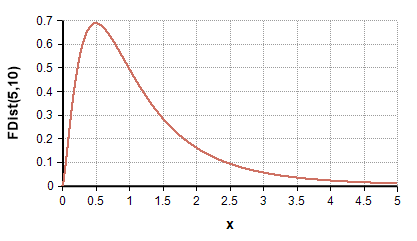# F-distribution

Release: 4.6  •  5.0  •  5.1  •  5.2  •  5.3  •  5.4  •  6.0  •  6.1  •  6.2  •  6.3  •  6.4

The F-distribution is used in classical statistics for hypothesis testing involving the comparison of variances between two samples (ANOVA = ANalysis Of VAriance), or for testing whether one model (such as a regression fit) is statistically superior to another. In also goes by the names Snedecor's distribution and the Fisher-Snedecor distribution.The F-distribution is a positive-only distribution

The ratio of two random variates each having a ChiSquared distribution has a F-distribution.

## Functions

### FDist(dof1, dof2)

New to Analytica 5.2

The distribution function.

### DensFDist(x, dof1, dof2)

The Analytic density function. Returns the probability density at «x» of an F-distribution with «dof1» and «dof2» degrees of freedom.

### CumFDist(x, dof1, dof2)

The cumulative density at «x» for the F-distribution with degrees of freedom «dof1» and «dof2». This is the area under the probability density graph falling to the left of «x».

### CumFDistInv(p, dof1, dof2)

The inverse cumulative density function (quantile function) of a F-distribution with «dof1» and «dof2» degrees of freedom. Returns the pth fractile/quantile/percentile.

## Statistics

• Mean = dof2 / (dof2 - 2), when dof2>2
• Mode =
• Variance =

## F-tests

### Are means of two normal distributions equal?

When they are assumed to have the same standard deviation.... (TBD)

(TBD)

(TBD)

(TBD)